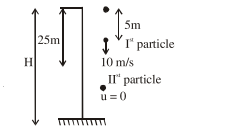# A stone is dropped from the top of a building.`
Question:

A stone is dropped from the top of a building. When it crosses a point $5 \mathrm{~m}$ below the top, another stone starts to fall from a point $25 \mathrm{~m}$ below the top. Both stones reach the bottom of building simultaneously. The height of the building is :

1. $35 \mathrm{~m}$

2.  $45 \mathrm{~m}$

3. $50 \mathrm{~m}$

4. $25 \mathrm{~m}$

Correct Option: , 2

Solution:Time for particle to meet $=\mathrm{t}^{\prime}=\frac{\mathrm{S}_{\mathrm{rel}}}{\mathrm{S}_{\mathrm{rel}}}=\frac{20}{10}=2 \mathrm{sec}$

Time taken by Ist particle to reach ground $=3 \mathrm{sec}$

$\mathrm{H}=\frac{1}{2} \mathrm{~g}(3)^{2}=45 \mathrm{~m}$